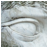SUM UNIVERSAL®Copying is slavery, you have to reach the spirit.

~ Zen Proverb

 All living souls welcome whatever they are ready to cope with; all else they ignore, or pronounce to be monstrous and wrong, or deny to be possible. ~ George Santayana, philosopher (1863-1952)

 You are a child of God. Your playing small does not serve the world. There is nothing enlightening about shrinking, so that other people won’t feel insecure around you. We are born to make manifest the glory of God that is within us. It is not just in some of us; it is in everyone. And as we let our light shine, we unconsciously give other people permission to do the same. As we are liberated from our own fear, our presence automatically liberates others. ~ Marianne Williamson, spiritual activist, lecturer, and author (1952-)

HOME

NUMBERS

NUMBER Pi

PATTERNS

SERVICES

WORKSHOPSReflections ’Energetic Fields – Common Denominators’

Before the election of 2016 is over, I would like to express some thoughts. As with everything else, the vibrational energy of numbers also plays a role in the lives of the presidents of the United States of America. The additional vibrational energy hovering over the President during the presidency is mostly clear, and also limited due to the organizations, missions, and personnel assisting him, or her in the daily work activities. Numbers in the President’s numerological profile will be amplified when they also appear predominantly in the surrounding energetic field with which the President is in close work relations.

To get a clearer picture for specific settings with their associated numbers and influential energies, we have to be very clear about the constituents of these settings, including being consistent with their names. Though we often use abbreviated names, we need to keep in mind that the all-pervading vibrational energy for the whole specific entity is always present. Therefore I use the whole name in my considerations when contemplating about the influential energetic field of numbers in certain settings.

Given these considerations, I consider the following vibrationally energetic settings to be of utmost importance for the President of the United States: United States of America (name of country), Congress of the United States of America (parties with whom he is in constant interaction and discourse), Supreme Court of the United States of America (selection of judiciary officials), World (as well as taking on the responsibility of foreign affairs).

Since the energetic settings are established, we now can see which numbers will play additionally an important role in the life of the President. And vice versa, since we know the numerological numbers of the President, we can see which numbers will add an oomph to the amplification of these energetic entity settings.

Now, here are the numbers that are very relevant for these four energetic entity settings:
The numerical equivalent for the name of the country is number three: [United States of America: U=21/3 (2+1=3), N=14/5 (1+4=5), I=9, T=20/2 (2+0=2), E=5, D=4, S=19/1 (1+9=10 and 1+0=1), T=20/2 (2+0=2), A=1, T=20/2 (2+0=2), E=5, S=19/1 (1+9=10 and 1+0=1), O=15/6 (1+5=6), F=6, A=1, M=13/4 (1+3=4), E=5, R=18/9 (1+8=9), I=9, C=3, A=1 and
3+5+9+2+5+4+1+2+1+2+5+1+6+6+1+4+5+9+9+3+1=84 and 8+4=12 and 1+3=3].
The numerical equivalent for the energetic field of important parties while in office is number four: [Congress of the United States of America: C=3, O=15/6 (1+5=6), N=14/5 (1+4=5), G=7, R=18/9 (1+8=9), E=5, S=19/1 (1+9=10 and 1+0=1), S=19/1 (1+9=10 and 1+0=1), O=15/6 (1+5=6), F=6, T=20/2 (2+0=2),H=8,E=5, U=21/3 (2+1=3),N=14/5 (1+4=5),I=9,T=20/2 (2+0=2),E=5,D=4, S=19/1 (1+9=10 and 1+0=1),T=20/2 (2+0=2), A=1,T=20/2 (2+0=2), E=5, S=19/1 (1+9=10 and 1+0=1), O=15/6 (1+5=6), F=6, A=1,M=13/4 (1+3=4), E=5, R=18/9 (1+8=9), I=9,C=3, A=1 and
3+6+5+7+9+5+1+1+6+6+2+8+5+3+5+9+2+5+4+1+2+1+2+5+1+6+6+1+4+5+9+9+3+1=148 and
1+4+8=13 and 1+3=4].
The numerical equivalent for a very strategic energetic field of great influence is number six: [Supreme Court of the United States of America: S=19/1 (1+9=10 and 1+0=1), U=21/3 (2+1=3), P=16/7 (1+6=7), R=18/9 (1+8=9), E=5, M=13/4 (1+3=4), E=5, C=3, O=15/6 (1+5=6), U=21/3 (2+1=3), R=18/9 (1+8=9), T=20/2 (2+0=2), O=15/6 (1+5=6), F=6, T=20/2 (2+0=2), H=8, E=5, U=21/3 (2+1=3), N=14/5 (1+4=5), I=9, T=20/2 (2+0=2), E=5, D=4, S=19/1 (1+9=10 and 1+0=1), T=20/2 (2+0=2), A=1, T=20/2 (2+0=2), E=5,S=19/1 (1+9=10 and 1+0=1), O=15/6 (1+5=6), F=6, A=1, M=13/4 (1+3=4), E=5, R=18/9 (1+8=9), I=9, C=3, A=1 and
1+3+7+9+5+4+5+3+6+3+9+2+6+6+2+8+5+3+5+9+2+5+4+1+2+1+2+5+1+6+6+1+4+5+9+9+3+1=168 and
1+6+8=15 and 1+5=6].
And not to forget the very important responsibility of foreign affairs which pertains to the ‘World’ with the vibrational energetic field of number nine: [WORLD: W=23/5 (2+3=5), O15/6 (1+5=6), R=18/9 (1+8=9), L=12/3 (1+2=3), D=4 and 5+6+9+3+4=27 and 2+7=9].

In conclusion of my thoughts, I like to state here that we can see, if we look at these settings from this perspective, which additional common denominators will play also an important role in this intricate and complex setup of state and world affairs. Since I have already written a lot about numbers, please read these articles to better understand the energetic properties of these numbers.

© Oct 22, 2016 Ute Sonja Medley (SUM UNIVERSAL)

Whenever we proceed from the known into the unknown we may hope to understand, but we may have to learn at the same time a new meaning of the word "understanding."

~ Werner Heisenberg